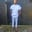Related Tags

communitycreator

# What is a Haskell function?Chinweuba Elijah Azubuike

### Overview

Haskell’s is a purely functional language and functions are its primary aspect.

Haskell syntax for declaring functions is as follows:

1. Function name
2. List of arguments
3. Outputs

The function definition is where you place the logic of the function.

### Code

Let’s see the syntax in the code below.

multiplication :: Integer -> Integer -> Integer   --function Instantiation
multiplication a b =  a * b                       --function definition/logic

addition :: Integer -> Integer -> Integer   --function Instantiation
addition d f =  d + f

main = do

print(multiplication 3 9)    --calling the function
print(addition 3 9)

### Explanation

• In the example above, we instantiate our function in the first line and define it in the second line.

• Next, we place the main logic of the function. In other words, we have written that the function takes two arguments and produces one integer type output.

• We did the same for the second function, addition, you will notice that when printing the result of the multiplication and addition function, they both work. This shows that the addition function will run without affecting the multiplication function unless the addition function throws an error while executing.

• The code will start executing from the main method.

To see the output, run the code above.

RELATED TAGS

communitycreator

CONTRIBUTORChinweuba Elijah Azubuike
RELATED COURSES

View all Courses

Keep Exploring

Learn in-demand tech skills in half the time